Question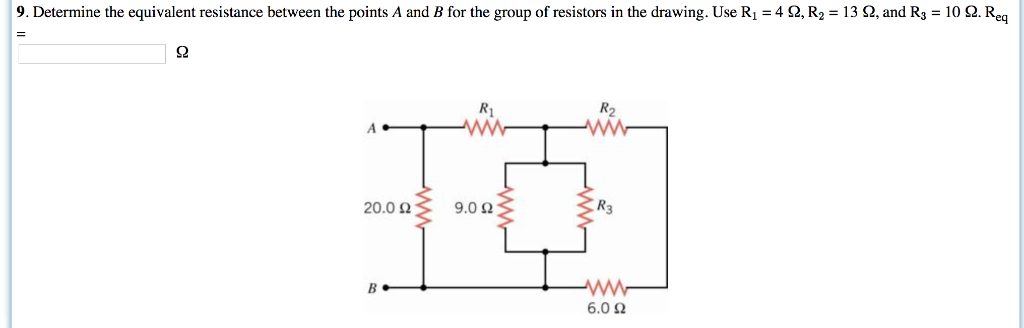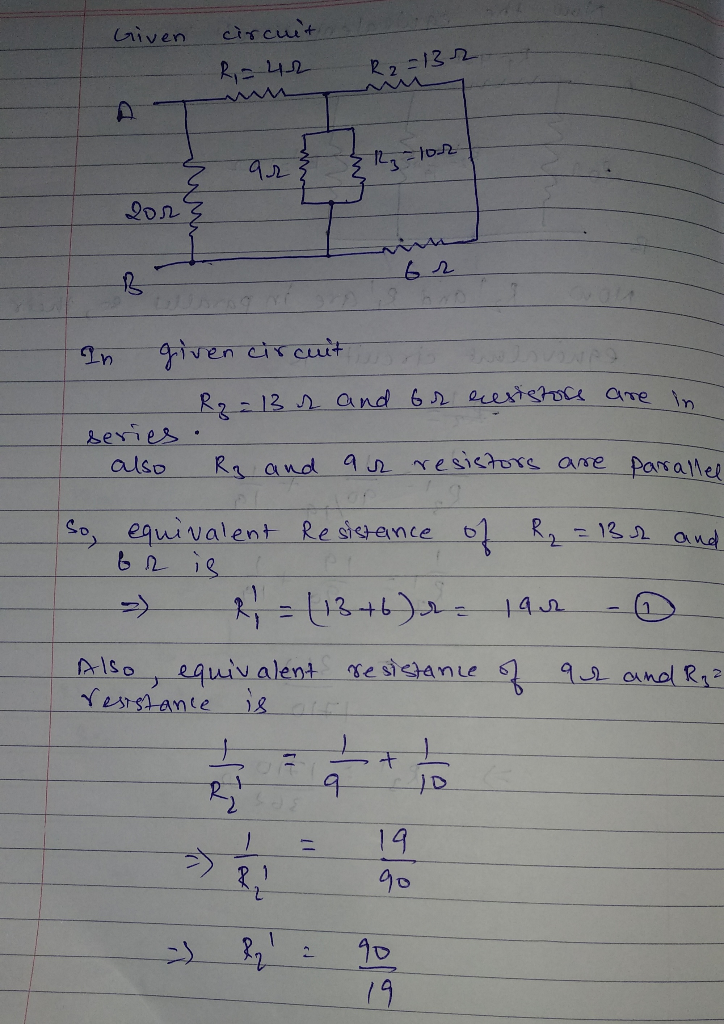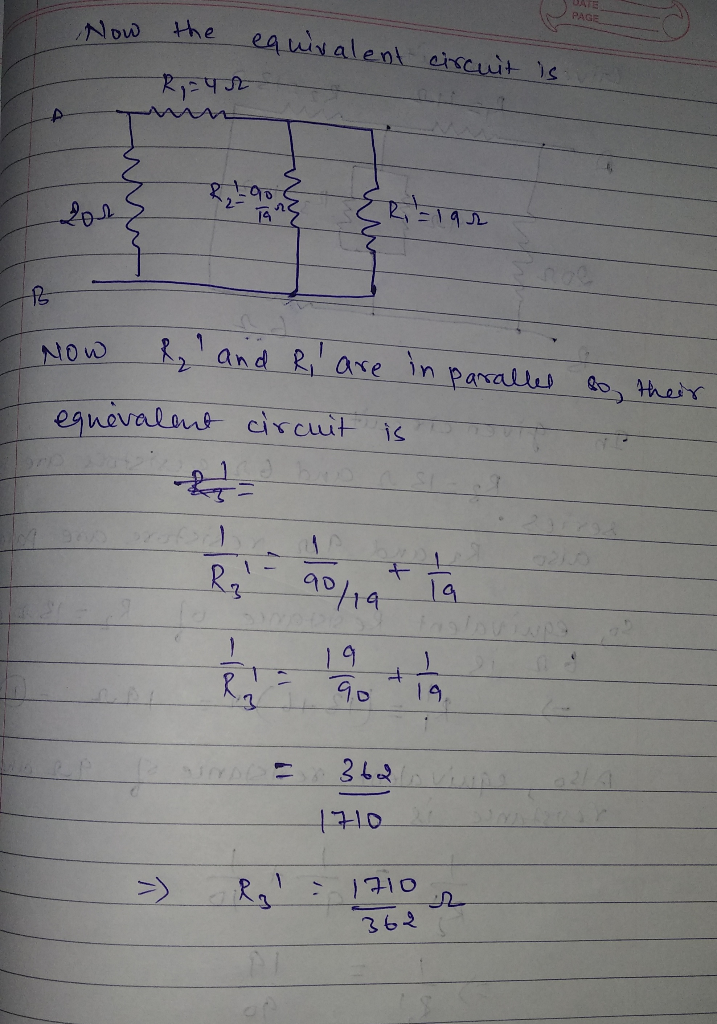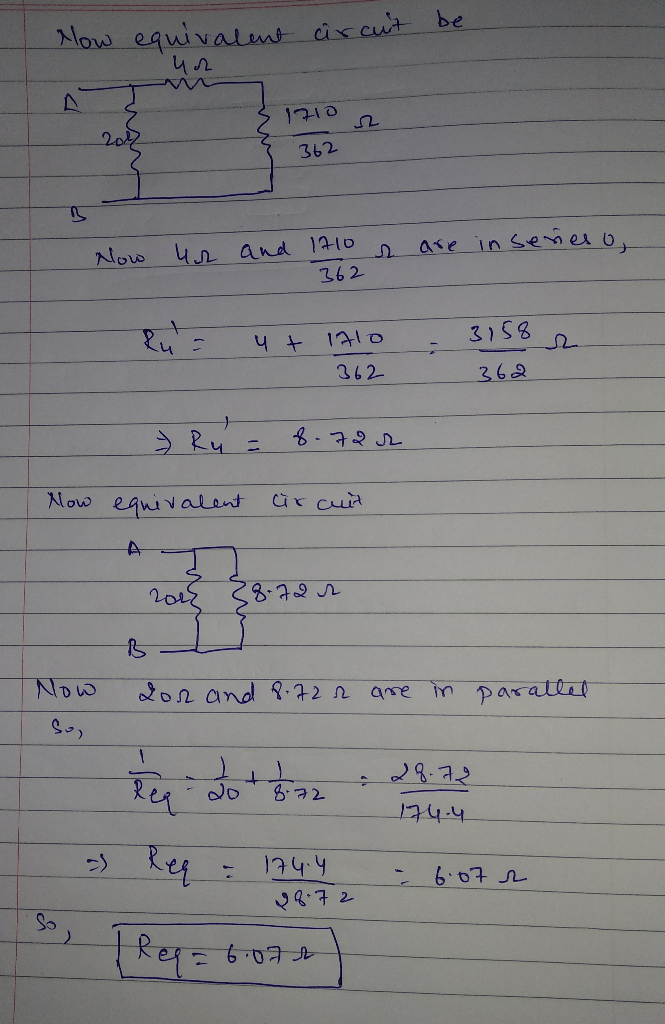#### Earn Coins

Coins can be redeemed for fabulous gifts.

Similar Homework Help Questions
• ### 65) Determine the equivalent resistance between the points A and B for the group of resistors...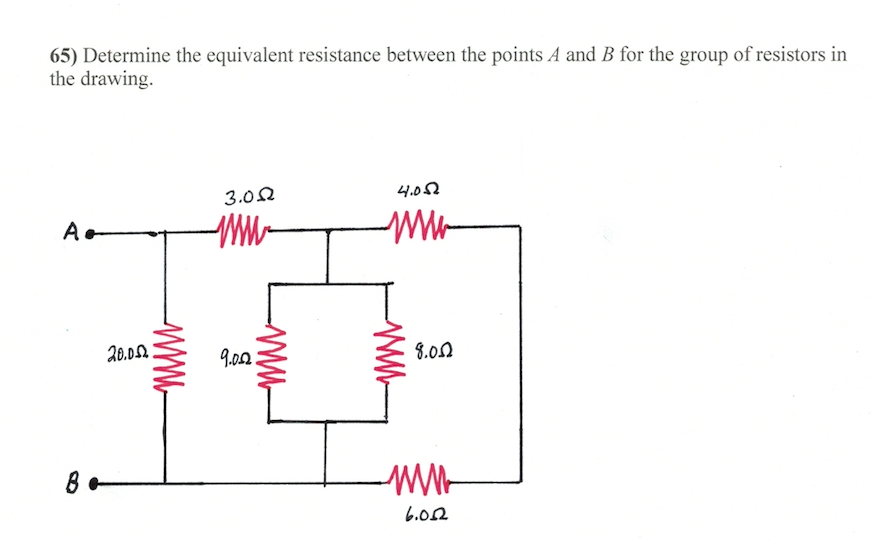65) Determine the equivalent resistance between the points A and B for the group of resistors in the drawing. 3.0 Ω 4.0 Ω Xo.DL 9.0Ω 3,0Ω .02

• ### Find the equivalent resistance of the combination of resistors shown above.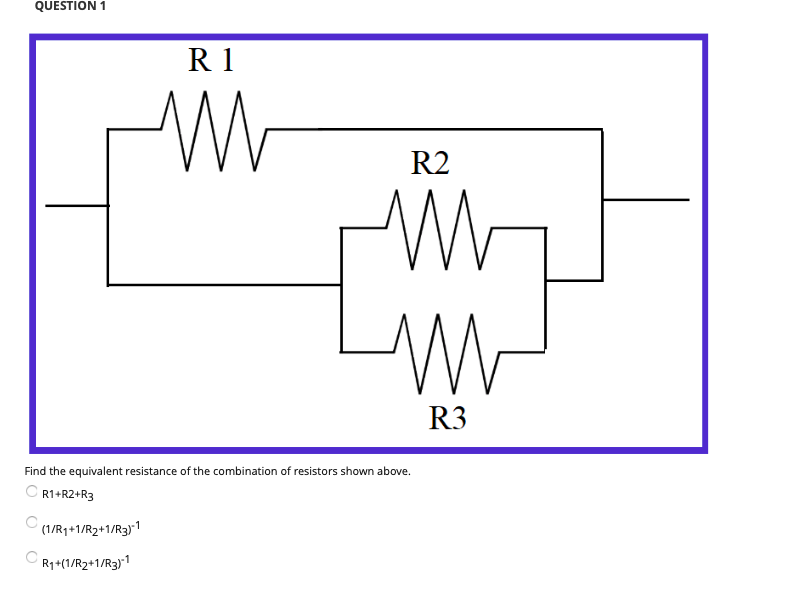Find the equivalent resistance of the combination of resistors shown above.R 1+R 2+R3(1 / R1+1 / R2+1 / R3)^{-1}R1+(1 / R2+1 / R3)^{-1}Find the equivalent resistance of the resistors shown above.(1 / R1+1 / R2)^{-1}+R3+R4R1+R2+(1 / R3+1 / R4)^{-1}R1+R2+R3+R4Assume the equivalent resistance in question 1 was 10 Ω and the equivalent resistance in question 2 was 40 Ω, and the same resistors were used for this new system. Determine the equivalent resistance of this system.

• ### What is the equivalent resistance of each group of resistors shown in the figure below? In...

What is the equivalent resistance of each group of resistors shown in the figure below? In the figure, R1 = 2.0 Ω, R2 = 3.0 Ω, R3 = 8.0 Ω, and R4 = 4.0 Ω. There is no figures present just Group A is in a series with R1 and R2 and R3. The secound Group is in a series with R4 and R4 and R4.

• ### 9. In this section of a circuit, resistors Ri, Ro, and Rs combine for an equivalent...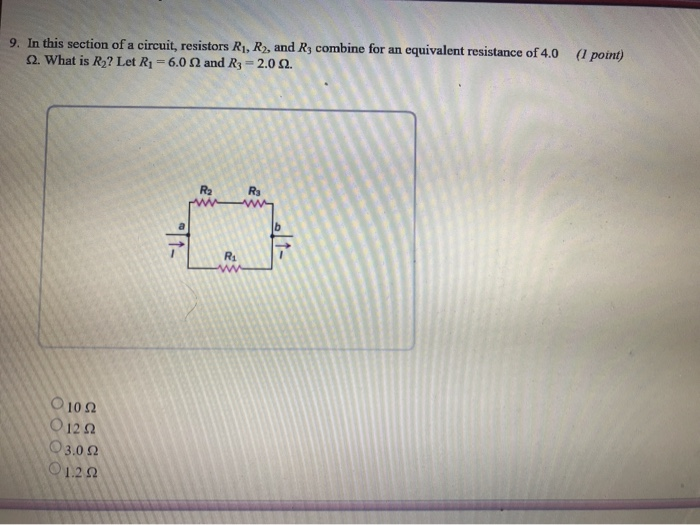9. In this section of a circuit, resistors Ri, Ro, and Rs combine for an equivalent resistance of 4.0 Ω. What is R2? Let R1-60 Ω and R3-20 Ω. ( point) Ri 10Ω 012Ω 3.0 Ω 1.2Ω 8. Three resistors are connected into the section of a circuit described by the diagram. Then the (I point) wire is cut at point t, and the two cut ends of the wire are separated. Through which of the three resistors, if any,...

• ### Question 3. (10 points) Four resistors are connected as shown in Figure 28.9a Find the equivalent resistance between a and c. R1-16 and R4-12 Ω R2-13 R3-4 (a) (b) What is the current in each resistor...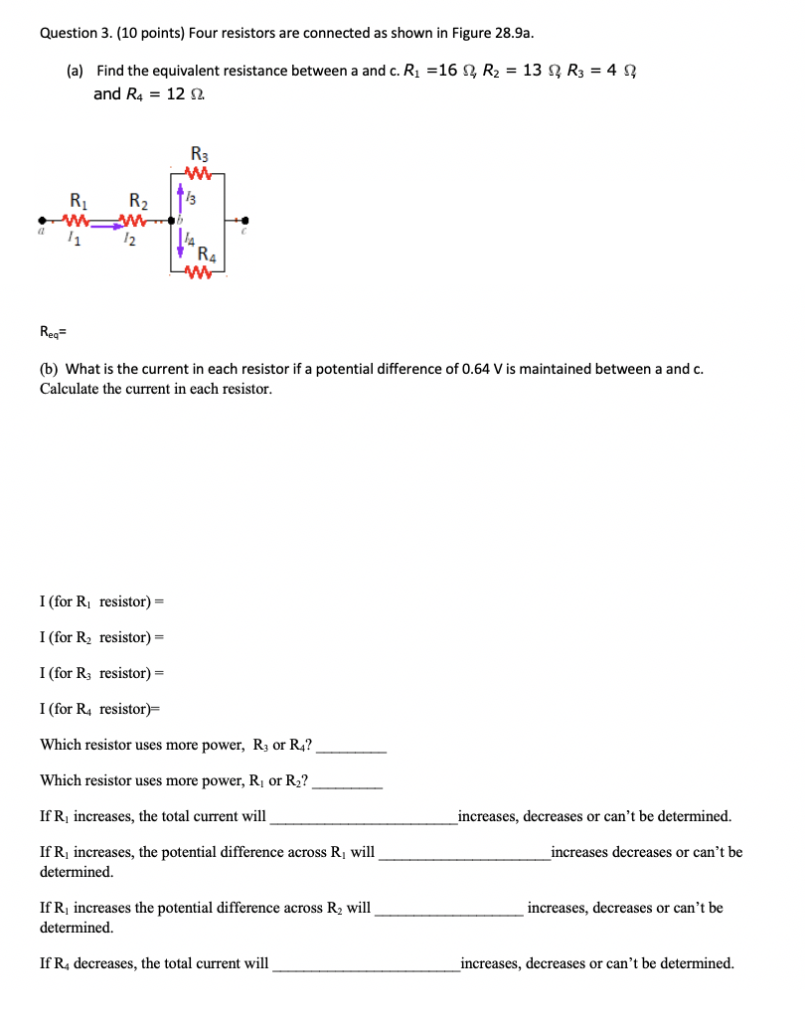Question 3. (10 points) Four resistors are connected as shown in Figure 28.9a Find the equivalent resistance between a and c. R1-16 and R4-12 Ω R2-13 R3-4 (a) (b) What is the current in each resistor if a potential difference of 0.64 V is maintained between a and c. Calculate the current in each resistor I (for Ri resistor)- I (for R2 resistor)- I (for R3 resistor) I (for R4 resistor)- Which resistor uses more power, R or R4? Which...

• ### What is the equivalent resistance of a the following 3 resistors: R1 = 13 Ohms R2...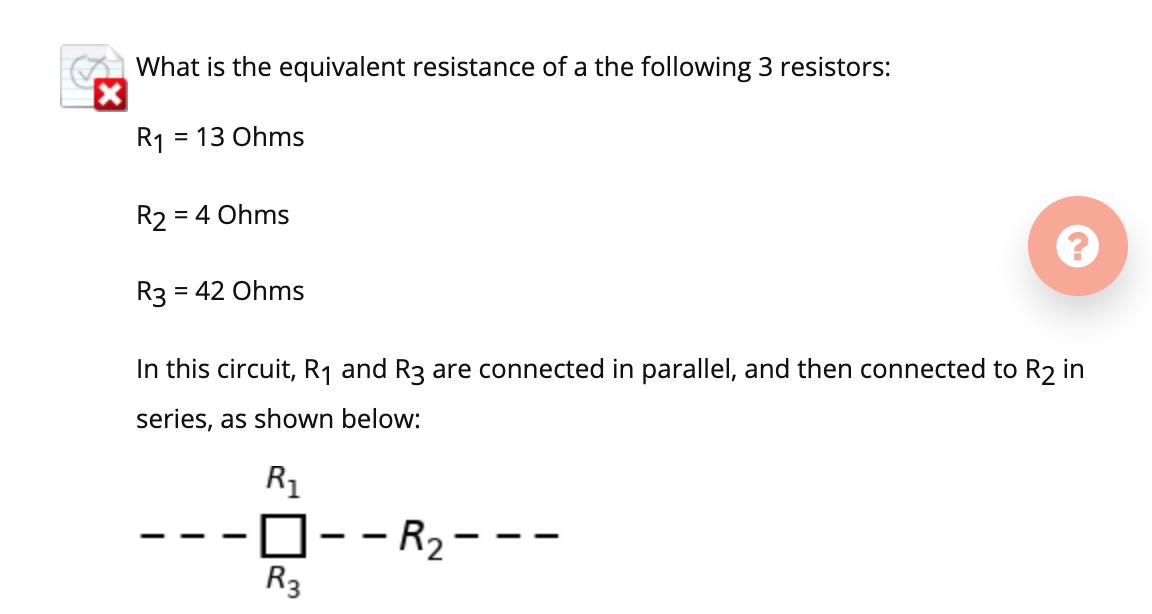What is the equivalent resistance of a the following 3 resistors: R1 = 13 Ohms R2 = = 4 Ohms R3 = 42 Ohms In this circuit, R1 and R3 are connected in parallel, and then connected to R2 in series, as shown below: Ri 0--R2- R3

• ### The equivalent resistance between points A and B of the circuit shown is 25.2 Ω. For...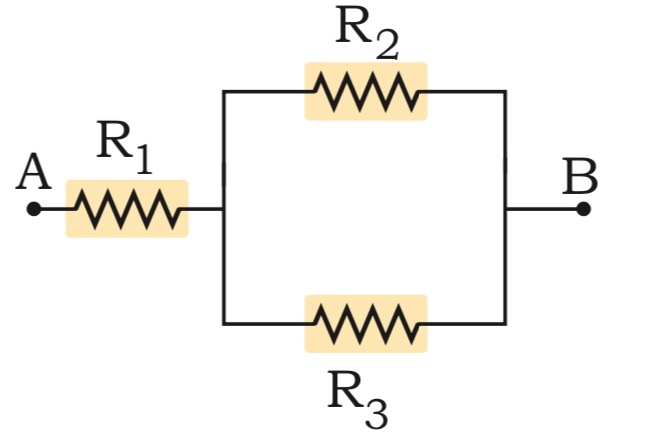The equivalent resistance between points A and B of the circuit shown is 25.2 Ω. For R1 = 10.3 Ω and R2 = 52.8 Ω, calculate the value of resistance R3. A B 1

• ### Consider the three resistors R1=11 Ω, R2=29 Ω, and R3=77 Ω in the configuration shown in the figure. A potential difference ΔV=3.5 V is applied between A and B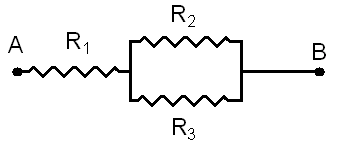Consider the three resistors R1=11 Ω, R2=29 Ω, and R3=77 Ω in the configuration shown in the figure. A potential difference ΔV=3.5 V is applied between A and B Part (a) Express the equivalent resistance Re of the combination of R2 and R3 in terms of R2 and R3.Part (b) Express the total resistance R off the combination of all three resistors in terms of R1, R2 and R3Part (c) Calculate the numerical value ofthe total resistance R in Ω.Part (d)...

• ### 4. Find the equivalent resistance between points A and B in the drawing (R1 = 4.0...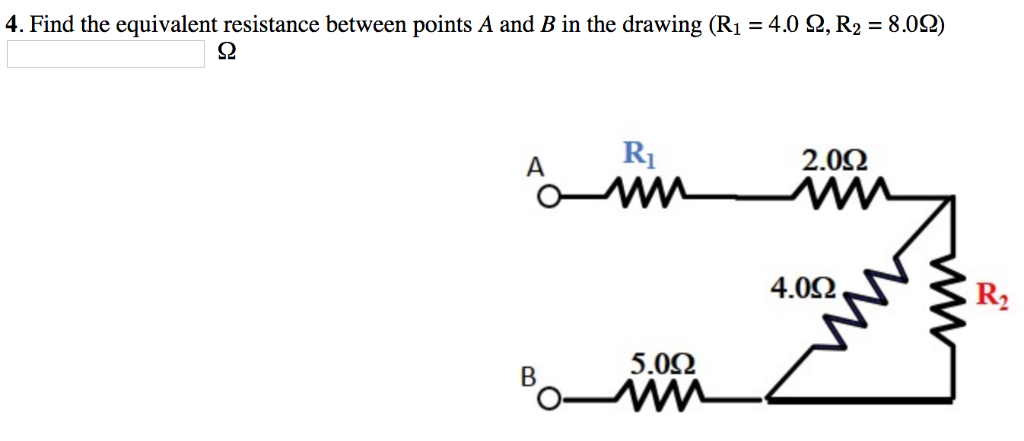4. Find the equivalent resistance between points A and B in the drawing (R1 = 4.0 Ω, R2 = 8.0Ω) S2 2.0Ω 4.0Ω R2 5.0Ω

• ### Find the equivalent resistance between points A and B in the drawing. 3.0 4.0 A, a...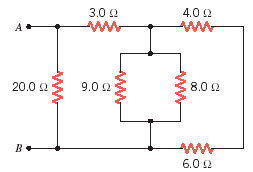Find the equivalent resistance between points A and B in the drawing. 3.0 4.0 A, a WW WW 20.0 9.0 2 8.0 6.0